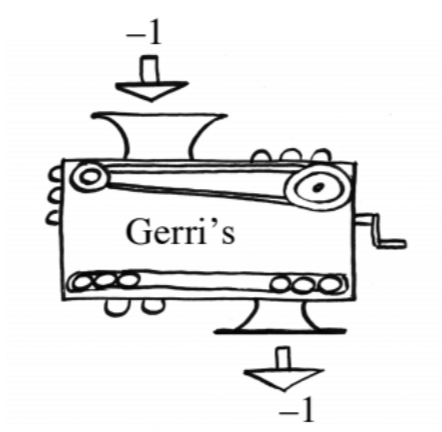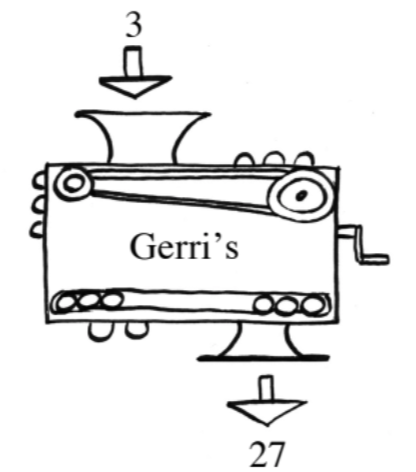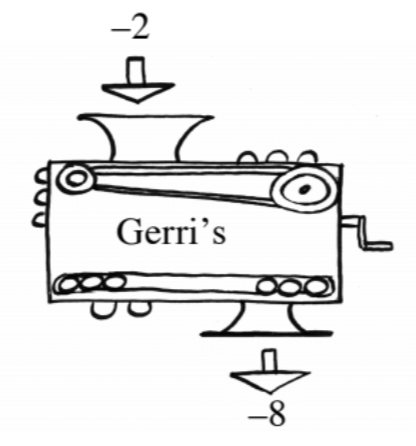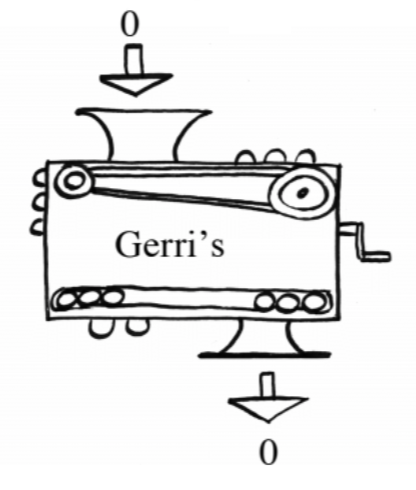### Home > INT3 > Chapter Ch1 > Lesson 1.1.2 > Problem1-26

1-26.

Gerri made a function machine. Below are four pictures of her machine. What is the equation for Gerri’s function machine?Make a table.

Notice that this is not a linear function because the rate of change is different between each pair.

Look for a pattern between all of the points. (Take a guess and then check.)

$f(x)=x^3$